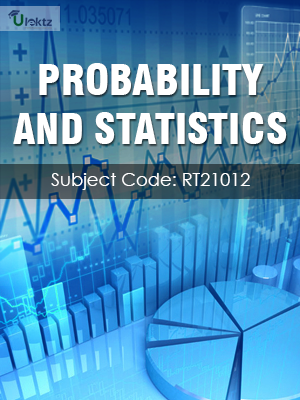•My WalletMy Order
•My Profile
•My Connections
•My Books
•My Videos
•My Tests
•My Calender
•My Messages
•My Shopping Cart
•My Orders
•Account Settings
•Help

# Book Details# Probability and Statistics

 Course Code : RT21012 Author : uLektz University : JNTU Kakinada Regulation : 2013 Categories : Civil Format :ePUB3 (DRM Protected) Type : eBook

FREE

Description :Probability and Statistics of RT21012 covers the latest syllabus prescribed by JNTU Kakinada for regulation 2013. Author: uLektz, Published by uLektz Learning Solutions Private Limited.

Note : No printed book. Only ebook. Access eBook using uLektz apps for Android, iOS and Windows Desktop PC.

##### Topics
###### Unit I Random variables and Distributions:

1.1 Introduction- Random variables

1.2 Distribution function

1.3 Discrete distributions (Review of Binomial and Poisson distributions)

1.4 Continuous distributions: Normal, Normal approximation to Binomial distribution, Gamma and Weibull distributions

###### Unit II Moments and Generating functions:

2.1 Introduction-Mathematical expectation and properties

2.2 Moment generating function, Moments of standard distributions (Binomial, Poisson and Normal distributions) – Properties

###### Unit III Sampling Theory:

3.1 Introduction - Population and samples

3.2 Sampling distribution of mean for large and small samples (with known and unknown variance)

3.3 Proportion sums and differences of means -Sampling distribution of variance -Point and interval estimators for means and proportions

###### Unit IV Tests of Hypothesis:

4.1 Introduction - Type I and Type II errors

4.2 Maximum error - One tail, two-tail tests

4.3 Tests concerning one mean and proportion

4.4 Two means- Proportions and their differences using Z-test, Student’s t-test - F-test and Chi -square test

4.5 ANOVA for one-way and two-way classified data

###### Unit V Curve fitting and Correlation:

5.1 Introduction - Fitting a straight line

5.2 Second degree curve-exponential curve-power curve by method of least squares

5.3 Simple Correlation and Regression

5.4 Rank correlation - Multiple regression

###### Unit VI Statistical Quality Control Methods:

6.1 Introduction - Methods for preparing control charts

6.2 Problems using x-bar, p, R charts and attribute charts

### Related Books